# Establishing the Equation of the Wave Function and the System Model (ρ, m, v)

Mohamed Daris*

Department of Mathematical Physics, University Rabbat Morocco, Morocco

Received Date: April 17,2019;  Published Date: April 26, 2019

#### Abstract

In this section a relation which establishes universal parameters of the matter will give us a new model that I call them the model (ρ, m, v) but in this model I will put relations between the parameters of the matter and the wave function and I’m going to explain that they are linking together the wave function plays the role of the wave function of matter and energy at the same time this gives us access to a new aspect that is a relationship of the wave function with mass and density makes it easier for us to exploit other relationships for the new model.

Keywords: New acceleration of light; Infinite spaces; Energy; Mass; Density; Full vacuum; Wave functions

Abbreviations: M: Mass; V: Volume; ρ: Density; E: Energy; C: Speed of Light; γ: New speed of Light; Ψ: Wave Function; TF : Time of the Universe

#### Introduction

The main purpose of this topic is to better understand new aspects of matter and energetic systems and to give new theorems and relations based on having an idea about the behaviour of matter either on the mass side of the volume or the volume density this gives us newly exploit relationships but on the basis of the corrective theorem of the equation of light and that will guide us to have an explanatory model of the material and hence the systems outside the vacuum or inside the void.

#### Discussion

Establishing the equation of the wave function and the system model (ρ, m, v):

##### Realization of the equations necessary to establish the density the volume the mass and assembly of the system (ρ, m, v):And we have the following two relationships: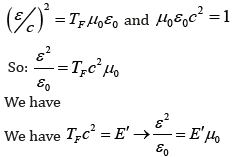So we have the following relationship: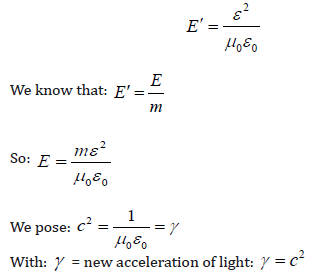With: γ = new acceleration of light: γ = c2

and : mε2′2

So we established the following new relationship:

E′ =γε′2

This is the new equation of light in infinite spaces condensed by emptiness full with energy E’.

E’: the maximum energy of the particle of matter in the full vacuum.

γ : is relative with acceleration.

ε ′ : is relative with the final time so we have the following new relationship:

E′ =γε ′2

##### Relationship building wave functions and speed C: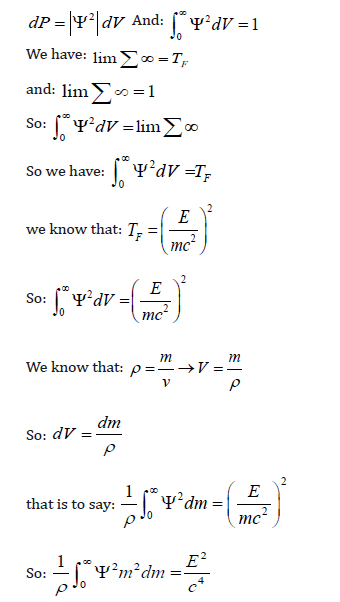→ So we will have the final result: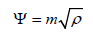→ this equation is the equation of condensed matter in infinite spaces of full energy.

##### Final relations of the worksSo we have the following new model (ρ ,m,v) :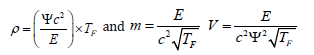These last results show that any material with a mass and a volume and a density tends towards a moment F T . That is to say that matter when it arrives at the moment F T its basic elements, which constitute them, mean that the volume and density disappear, and the matter no longer exists.

None.

#### Conflict of Interest

No conflict of interest.

Article Details
Citation
Keywords
Scroll to
Scroll to Top